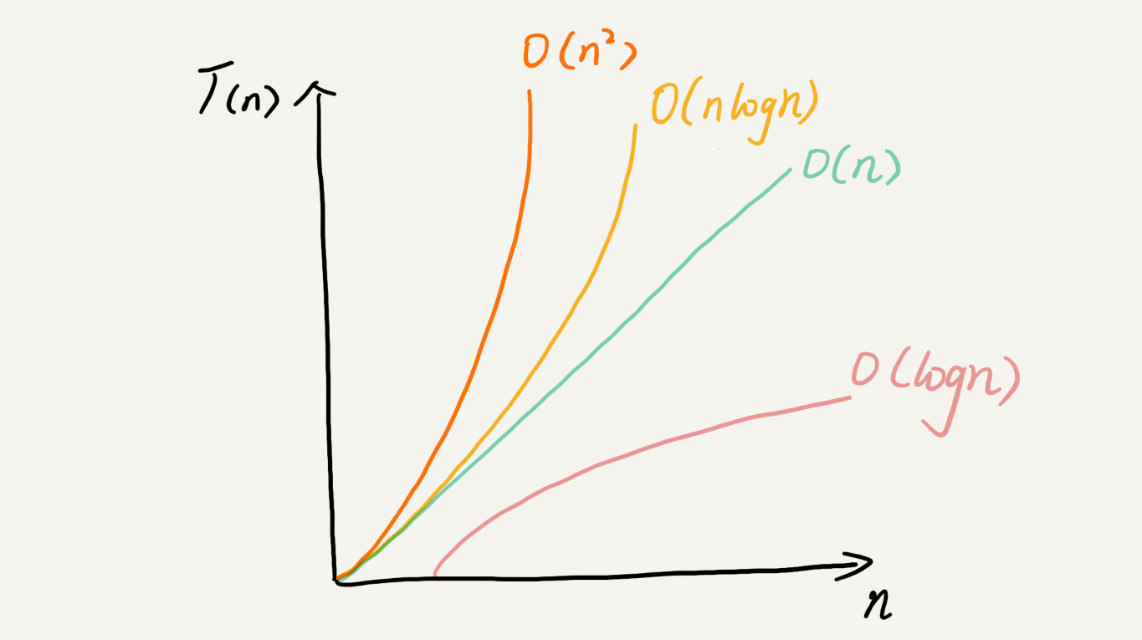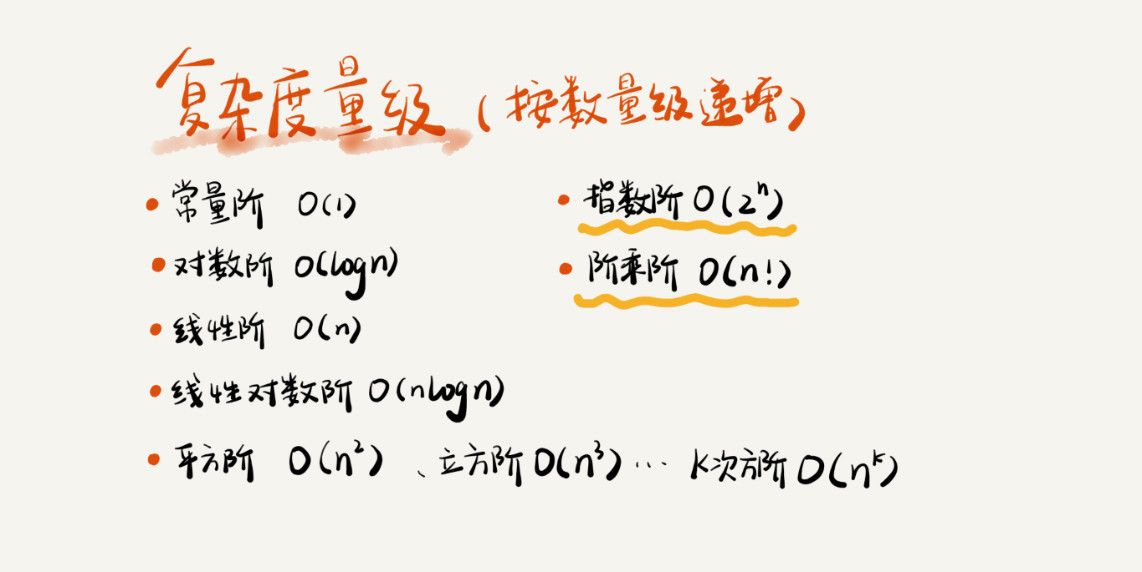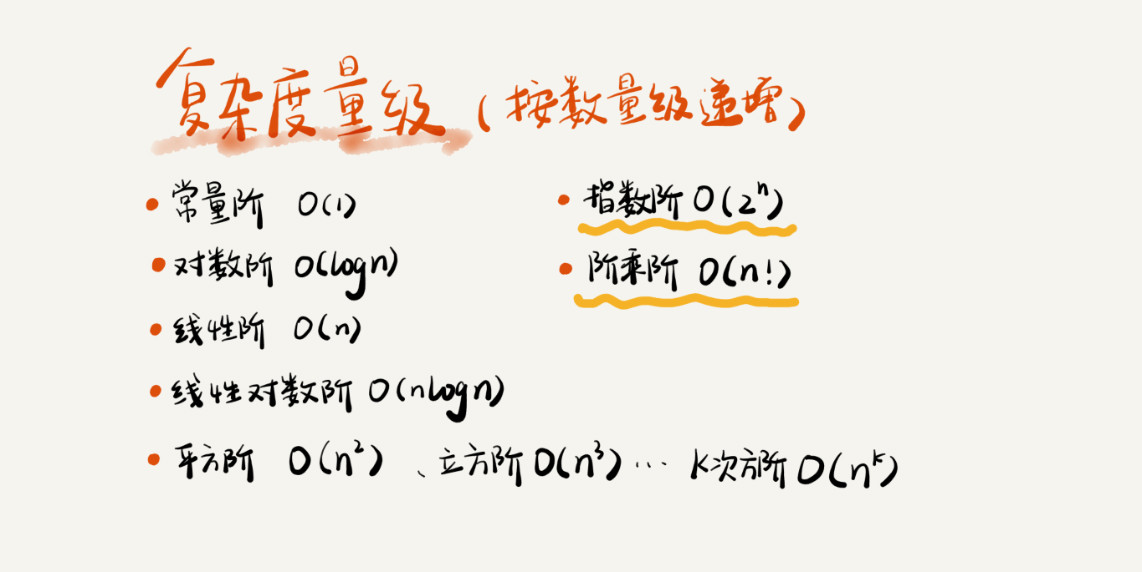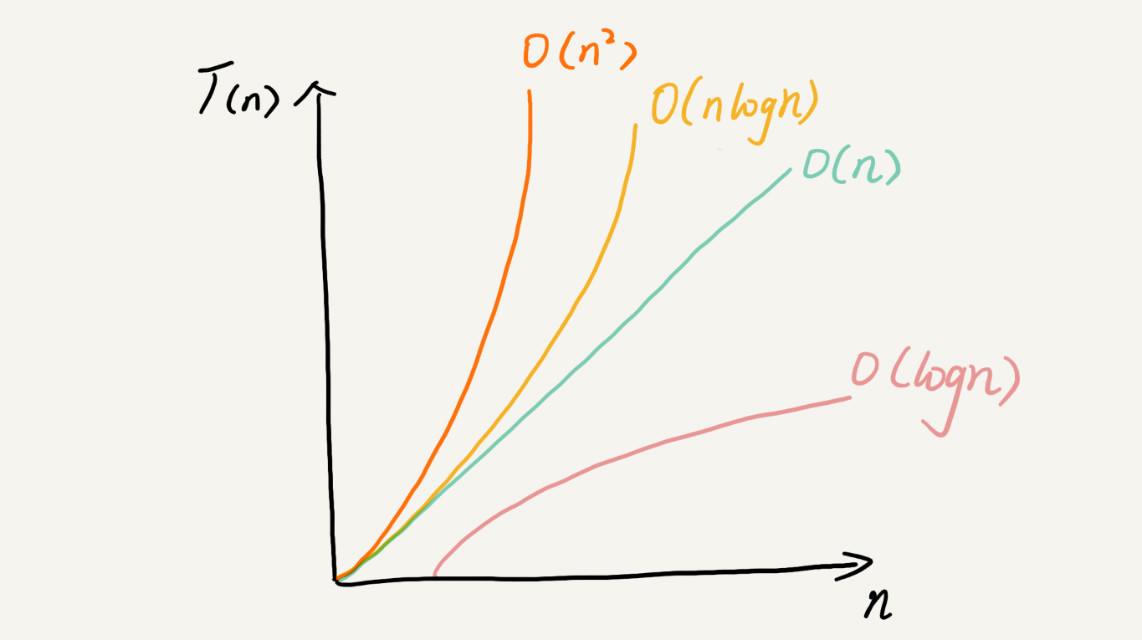• 如何让代码运行的更快，如何更省空间个重要的标准：复杂度分析 大 O 复杂度表示法 算法的执行效率，粗略的讲就是算法代码执行的时间，在我们不做精确监控统计，靠观察怎么分析代码执行的时间呢？ int cal(int ...最近练习算法题，又看了极客时间中的《数据结构与算法之美》写的真不错，于是总结一下关于复杂度的知识，代码和图片都是课程里面的。虽然是按课程写的，但是自己写一遍最好，否则看过就忘了。

数据结构与算法本身解决的是的问题。如何让代码运行的更快，如何更省空间有个重要的标准：复杂度分析

一、大 O 复杂度表示法

算法的执行效率，粗略的讲就是算法代码执行的时间，在我们不做精确监控统计，靠观察怎么分析代码执行的时间呢？

int cal(int n) {
int sum = 0;--------2
int i = 1;--------3
for (; i <= n; ++i) {--------4
sum = sum + i;--------5
}
return sum;
}

上面这段代码是计算1到n的累加和，从CPU的角度来看每一行的执行都是：读数据-计算-写数据。尽管每行代码对应的CPU执行时间都不一样，做粗略计算的时候只需要估算，把这个时间看成相同的一个时间：unit_time。

2，3行代码分别需要1*unit_time，for循环里面的第4,5行需要n*unit_time，得到的结果是2*unit_time+2n*unit_time = (2n+2)*unit_time。得出一个结论：所有代码执行的时间T(n)与每行代码执行的时间unit_time成正比

按照这个方法看下面一段代码：

int cal(int n) {
int sum = 0;--------2
int i = 1;--------3
int j = 1;--------4
for (; i <= n; ++i) {--------5
j = 1;--------6
for (; j <= n; ++j) {--------7
sum = sum +  i * j;--------8
}
}
}

2,3,4分别行执行了1次总共3*unit_time，5,6行分别执行了n*unit_time，7,8行分别执行了n^2*unit_time，总共是(2n^2+2n+3)*unit_time.尽管不知道*unit_time的具体值，但还是得到那个规律：代码执行的时间T(n)与每行代码执行的次数f(n)成正比

总结一个公式就是：1.  T(n)表示代码执行时间
2. n是数据规模
3. f(n)是每行代码执行次数总和
4. O表示代码执行时间和代码执行总次数成正比

所以第一段代码的T(n) = O(2n+2)，第二段代码的T(n) = O(2n^2+2n+3)这就是时间复杂度（asymptotic time complexity）的表示方法

而代码规模n很大时就可以忽略掉低阶，常量和系数，最终得到，第一段代码的T(n) = O(n)，第二段T(n) = O(n^2)

二、时间复杂度分析

1.只关注循环执行次数最对的代码

就像上面第一段代码，我们只关注for循环的运行次数就可以得出这段代码的时间复杂度是T(n) = O(n)

2.加法法则：总复杂度等于量级最大的那段代码的复杂度

这里有段代码，我们分析一下

int cal(int n) {
int sum_1 = 0;
int p = 1;
for (; p < 100; ++p) {
sum_1 = sum_1 + p;
}

int sum_2 = 0;
int q = 1;
for (; q < n; ++q) {
sum_2 = sum_2 + q;
}

int sum_3 = 0;
int i = 1;
int j = 1;
for (; i <= n; ++i) {
j = 1;
for (; j <= n; ++j) {
sum_3 = sum_3 +  i * j;
}
}

return sum_1 + sum_2 + sum_3;
}

这段代码可以分为三部分sum_1、sum_2、sum_3

1. 第一部分for'循环了100次，时间复杂度是O(1)，常数级别的如果有更高阶可以忽略掉，为什么是O(1)下面解释
2. 第二部分的时间复杂度分别是O(n)
3. 第三部分的时间复杂度分别是O(n^2)

很显然最高阶是第三部分，所以这段代码的时间复杂度是O(n^2)

3.乘法法则：嵌套代码的复杂度等于嵌套内外代码复杂度的乘积

看一段代码

int cal(int n) {
int ret = 0;
int i = 1;
for (; i < n; ++i) {
ret = ret + f(i);
}
}

int f(int n) {
int sum = 0;
int i = 1;
for (; i < n; ++i) {
sum = sum + i;
}
return sum;
}

for循环里面嵌入的方法f(x)的时间复杂度为O(n)外部for循环的复杂度是O(n)那么这段代码的时间复杂度就是O(n*n)=O(n^2)。其实还是跟上面分析的一样是两个否循环的嵌套跟2中那个最高阶的一样。

三、常见的时间复杂度实例上面这些可以分两类，多项式量级非多项式量级，其中费多项式量级只有划黄色下划线那两个。非多项式量级的算法问题叫作 NP（Non-Deterministic Polynomial，非确定多项式）问题。

按我们的数学知识，非多项式量级的时间复杂度会随数据规模n的增大而急剧增大，求解问题的执行时间会无线增长，所以非多项式量级的算法其实效率非常低。所以不多去分析了，只分析多项式量级算法。

1.O(1)

看段代码

int i = 8;
int j = 6;
int sum = i + j;

这段代码的时间复杂度并不是O(3)而是O(1)，只要代码的运行时间不随n的增大而增长我们都记作O(1)，只要不存在递归和循环，再多行代码的时间复杂度都是O(1)

2. 对数阶O(logn)、O(nlogn)

i=1;
while (i <= n)  {
i = i * 2;
}

不难看出这段代码的第三行的阶数最高，i的取值是个等比数列x=如果把2换成3就有其实不管底是2，3还是10时间复杂度都可以记为O(logn)因为=（C * 其中C = 是个常数可以忽略，对数阶时间复杂度表示的时候可以省略调对数的底，统一写成O(logn)。理解了这个O(nlogn)其实就不难理解了，就是一个嵌套的乘法法则，一个循环嵌套一个时间复杂度为O(logn)的代码。比如归并排序和快速排序的时间复杂度都是O(nlogn)

3. O(m+n)、O(m*n)

代码的时间复杂度由两种数据的规模来决定

int cal(int m, int n) {
int sum_1 = 0;
int i = 1;
for (; i < m; ++i) {
sum_1 = sum_1 + i;
}

int sum_2 = 0;
int j = 1;
for (; j < n; ++j) {
sum_2 = sum_2 + j;
}

return sum_1 + sum_2;
}

像这种我们无法预估m和n的量级谁的比较大，所以无法用加法法则忽略掉一个，所以表示为O(m+n)，但乘法法则依然有效所以可以有O(m * n)

四、空间复杂度

空间复杂度的全称是渐进空间复杂度（asymptotic space complexity），表示的是算法的存储空间跟数据规模的增长关系。看代码：

void print(int n) {
int i = 0;
int[] a = new int[n];
for (i; i <n; ++i) {
a[i] = i * i;
}

for (i = n-1; i >= 0; --i) {
print out a[i]
}
}

代码中申请了一个长度为n的数组，其他地方没有占用到更多空间，所以空间复杂度就是O(n)，常见的空间复杂度就是 O(1)、O(n)、O(n2 )，像 O(logn)、O(nlogn) 这样的对数阶复杂度平时都用不到。而且，空间复杂度分析比时间复杂度分析要简单很多。

展开全文• 什么是数据结构和算法 复杂度分析 时间复杂度度 空间复杂度

什么是数据结构和算法

数据结构就是指一组数据的存储结构，算法就是操作数据的一组方法。
数据结构是为算法服务的，算法要作用在特定的数据结构上。数据结构是静态的，它只是组织数据的一种方式。如果不在它的基础上操作、构建算法，孤立存在的数据结构就是没用的。

复杂度分析

数据结构和算法解决的是如何更省、更快地存储和处理数据的问题，因此，我们就需要一个考量效率和资源消耗的方法，这就是复杂度分析方法。
很多时候我们受限于环境和数据量，没有办法通过运行程序的方式，计算代码的执行时间和占用内存的大小，这时候我们需要一种可以不依赖数据，就能估算出算法执行效率的方法。

大O复杂度表示法

算法的执行时间与每行代码的执行次数成正比，用T(n) = O(f(n))表示，其中T(n)表示算法执行总时间，f(n)表示每行代码执行总次数，而n往往表示数据的规模。
大 O 时间复杂度并非指代码真正的执行时间，而是表示代码执行时间随数据规模增长的变化趋势，所以，也叫作渐进时间复杂度（asymptotic time complexity），简称时间复杂度。公式中的低阶、常量、系数三部分并不左右增长趋势，所以都可以忽略。

时间复杂度

时间复杂度分析的方法：

1. 只关注循环次数最多的一段代码
2. 加法法则：总复杂度等于量级最大的那段代码的复杂度，比如有两段代码，一段代码复杂度是O(n)，另一段代码是O(2^n)，总复杂度就是 O(2^n)
3. 乘法法则：嵌套代码的复杂度等于嵌套内外代码复杂度的乘积

常见的复杂度量级：上述量级可以粗略分为，多项式量级和非多项式量级，其中非多项式量级的只有O(2^n) 和 O(n!)。
当n越来越大，非多项式量级的算法执行时间会急剧增加，所以它们是非常低效的方法。

多项式量级介绍：

1. O(1)：一般情况下，只要算法中不存在循环语句、递归语句，即使有成千上万行的代码，其时间复杂度也是Ο(1)
2. O(logn)、O(nlogn)：对数阶时间复杂度比较难分析，看下面一段代码
i=1;
while (i <= n)  {
i = i * 2;
}

i = i * 2 这行代码执行次数最多，所以只需要分析它的时间复杂度即可。从代码中可以看出，当 2^x = n 时结束循环， 计算 x=log2n，所以这段代码的时间复杂度就是 O(log2n)。

1. O(m+n)、O(m*n)：m 和 n 是表示两个数据规模。我们无法事先评估 m 和 n 谁的量级大，所以我们在表示复杂度的时候，就不能简单地利用加法法则，省略掉其中一个。所以，上面代码的时间复杂度就是 O(m+n)。

空间复杂度

空间复杂度全称就是渐进空间复杂度，表示算法的存储空间与数据规模之间的增长关系，通过下面一段代码分析空间复杂度

void print(int n) {
int i = 0;
int[] a = new int[n];
for (i; i <n; ++i) {
a[i] = i * i;
}

for (i = n-1; i >= 0; --i) {
print out a[i]
}
}

第 2 行代码int i = 0;，我们申请了一个空间存储变量 i，但是它是常量阶的，跟数据规模 n 没有关系，所以我们可以忽略。第 3 行 int[] a = new int[n] 申请了一个大小为 n 的 int 类型数组，除此之外，剩下的代码都没有占用更多的空间，所以整段代码的空间复杂度就是 O(n)。
我们常见的空间复杂度就是 O(1)、O(n)、O(n2 )，像 O(logn)、O(nlogn) 这样的对数阶复杂度平时都用不到。而且，空间复杂度分析比时间复杂度分析要简单很多。所以，对于空间复杂度，掌握刚我说的这些内容已经足够了。

参考：极客时间《数据结构与算法之美》课程

展开全文算法 数据结构 复杂度分析
• 知道了大O复杂度表示法的由来和表示方法，下面讲三个使用方法分析一段代码的时间复杂度。 只关注循环执行次数最多的一段代码 加法法则：总复杂度等于量级最大的那段代码的复杂度 乘法法则：嵌套代码的复杂度等于...

入门篇
基础篇
高级篇
实战篇

前言

复杂度分析是整个算法学习的精髓，只要掌握了它，数据结构和算法的内容基本上就掌握了一半。

一、大 O 复杂度表示法

大 O 复杂度表示法的作用是：在不运行代码的情况下，用“肉眼”得到一段代码的执行时间。

int cal(int n) {
int sum = 0;
int i = 1;
for (; i <= n; ++i) {
sum = sum + i;
}
return sum;
}

假设每一行的执行时间为Δt，那么第2、3行是 1Δt 。第4行和第5行分别执行了n次，所以第4行的执行时间是 nΔt，第五行也是 nΔt 。所以这段代码总执行时间T(n)：

T(n) = (2n + 2)Δt

可见，所有代码的执行时间 T(n) 与每行代码的执行次数成正比。

再来看嵌套循环的例子：

int cal(int n) {
int sum = 0;
int i = 1;
int j = 1;
for (; i <= n; ++i) {
j = 1;
for (; j <= n; ++j) {
sum = sum +  i * j;
}
}
}

外面的for循环执行了n次，最里面的for循环执行了 n² ，按照上面的分析方法：
1、2、3行分别执行了 1次，执行时间加起来就是3Δt；
5、6行都执行了n次，执行时间分别是 nΔt，加起来就是 2nΔt；
第7、8分别执行 n²次，加起来的时间就是 2n²Δt。行可知整段代码的总执行时间T(n)：

T(n) = (3 + 2n + 2n²) Δt

通过以上两个示例，我们发现规律： 所有代码的执行时间 T(n) 与每行代码的执行次数 f(n) 成正比。

由此引出大O复杂度表示法：

T(n) = O( f(n) )

• T(n) 表示代码执行时间；
• n 表示数据规模的大小
• f(n) 表示每行代码执行的次数总和。

二、时间复杂度分析

大 O时间复杂度实际上并不具体表示代码真正的执行时间，而是表示代码执行时间随数据规模增长的变化趋势，所以，也叫作渐进时间复杂度（asymptotic time complexity），简称时间复杂度。

知道了大O复杂度表示法的由来和表示方法，下面讲三个使用方法分析一段代码的时间复杂度。

1. 只关注循环执行次数最多的一段代码
2. 加法法则：总复杂度等于量级最大的那段代码的复杂度
3. 乘法法则：嵌套代码的复杂度等于嵌套内外代码复杂度的乘积

1. 只关注循环执行次数最多的一段代码
第一段代码示例中执行次数最多的是第4和5行，执行了n次，因此总的时间复杂度就是O(n)。

2. 加法法则：总复杂度等于量级最大的那段代码的复杂度

int cal(int n) {
int sum_1 = 0;
int p = 1;
for (; p < 100; ++p) {
sum_1 = sum_1 + p;
}

int sum_2 = 0;
int q = 1;
for (; q < n; ++q) {
sum_2 = sum_2 + q;
}

int sum_3 = 0;
int i = 1;
int j = 1;
for (; i <= n; ++i) {
j = 1;
for (; j <= n; ++j) {
sum_3 = sum_3 +  i * j;
}
}

return sum_1 + sum_2 + sum_3;
}

第一段执行了100次，但就算执行了10000次，只要和n没关系就忽略；第二段和第三段的时间复杂度分别是O(n) 和 O(n2)。综合这三段代码的时间复杂度，我们取其中最大的量级。所以，整段代码的时间复杂度就为 O(n2)。也就是说：总的时间复杂度就等于量级最大的那段代码的时间复杂度。

3. 乘法法则：嵌套代码的复杂度等于嵌套内外代码复杂度的乘积
上面嵌套循环的例子中，外层for循环的时间复杂度是O(n)，内层的时间复杂度其实是外层的O(n)乘以内层的O(n)，所以整个cal()函数的时间复杂度就是O(n²)。

几种常见时间复杂度实例分析指数阶和阶乘阶是非多项式。当数据规模 n 越来越大时，非多项式量级算法的执行时间会急剧增加，求解问题的执行时间会无限增长。所以，非多项式时间复杂度的算法其实是非常低效的算法。不讲。

1. O(1)

首先你必须明确一个概念，O(1) 只是常量级时间复杂度的一种表示方法，并不是指只执行了一行代码。比如这段代码，即便有 3 行，它的时间复杂度也是 O(1），而不是 O(3)。

int i = 8;
int j = 6;
int sum = i + j;

只要代码的执行时间不随 n 的增大而增长，这样代码的时间复杂度我们都记作 O(1)。或者说，一般情况下，只要算法中不存在循环语句、递归语句，即使有成千上万行的代码，其时间复杂度也是Ο(1)。

2. O(logn)、O(nlogn)

对数阶时间复杂度非常常见，同时也是最难分析的一种时间复杂度。我通过一个例子来说明一下。

i=1;
while (i <= n)  {
i = i * 2;
}

第三行代码是循环执行次数最多的。其实是等比数列。通过 2x=n 求解 x 这个问题我们想高中应该就学过了，我就不多说了。x=log2n，所以，这段代码的时间复杂度就是 O(log2n)。

其余的时间复杂度表达式就不写笔记了，极客时间的《数据结构和算法》强烈推荐。

空间复杂度分析

空间分析比较简单，我们常见的空间复杂度就是 O(1)、O(n)、O(n2 )。分析方法和时间复杂度一样，不再赘述。

总结

复杂度也叫渐进复杂度，包括时间复杂度和空间复杂度，用来分析算法执行效率与数据规模之间的增长关系，可以粗略地表示，越高阶复杂度的算法，执行效率越低。常见的复杂度并不多，从低阶到高阶有：O(1)、O(logn)、O(n)、O(nlogn)、O(n2 )。复杂度分析并不难，关键在于多练。
下一节说一下真实代码中的复杂度分析。

展开全文• 若将每条语句执行的单位时间看作一致的，也就将得出一个规律：一段代码的执行时间T(n)与每一条语句总的执行次数（累加数）成正比。即公式： T(n) = O(f(n)) 其中T(n)代表代码执行的总时间 ；n表示数据规模； f(n)...

（一）大O（字母大写O）复杂度表示法

若将每条语句执行的单位时间看作一致的，也就将得出一个规律：一段代码的执行时间T(n)与每一条语句总的执行次数（累加数）成正比。即公式：

T(n) = O(f(n))

其中T(n)代表代码执行的总时间 ；n表示数据规模； f(n)表示每条语句执行次数的累加和，此值与n密切相关；公式中的O表示T(n)f(n)成正比。
实际上，大O时间复杂度并不真正具体表示代码执行时间，而是表示代码执行时间随数据规模增大的变化趋势，因此也称为渐进时间复杂度（asymptotic time complexity），简称时间复杂度。
n很大时，公式中的低阶、常量、系数三部分并不左右增长趋势，因此可忽略。此时，只需记最大量级。形如：

T(n) = O(2n+3)     =>    T(n) = O(n)

注：非O(2n)， O表示的是变化趋势，而不是某一具体关系。

T(n) = O(2n^2 + 2n + 3)  =>  T(n) = O(n^2)

注：非O(n2 + n)，n2 >> n，故n被忽略。

（二）时间复杂度分析方法

1.加法法则

代码总的复杂度等于量级最大的那段代码的复杂度。
我们通常会忽略公式中的常量，低阶和系数只记录最大量级。
如下面一个例子：

int cal(int n) {
int sum_1 = 0;
int p = 1;
for(; p <= 100; ++p) {
sum_1 = sum_1 + p;
}

int sum_2 = 0int q = 1;
for(; q <= n; ++q) {
sum_2 = sum_2 + q;
}

int sum_3 = 0int i = 1int j = 1for(; i <= n; ++i) {
j = 1;
for(; j <= n; ++j) {
sum_3 = sum_3 + i * j;
}
}
return sum_1 + sum_2 + sum_3;
}

稍加分析即可得出，sum_1部分的执行时间为一个常量，sum_2部分时间复杂度为O(n)，sum_3部分的时间复杂度为O(n2)，最后的return句执行时间又是一个常量。

注：无论代码执行的次数多么巨大，即使是上亿次，只要执行时间是一个确定的值，那么它本身对于增长趋势没有任何影响，在大O法时间复杂度表示中总是被忽略。

由于这段代码是顺序执行的，其执行时间为各段代码执行时间之和，那么他的时间复杂度即取整段代码中量级最大的时间复杂度作为整段代码的时间复杂度，这段代码时间复杂度即为：

T(n) = O(n^2)

也就是说，代码总的时间复杂度等于量级最大的那段代码的时间复杂度，这就是加法法则，用公式表示即为：
若：

T1(n) = O(f(n));
T2(n) = O(g(n));

则：

T(n) = T1(n) + T2(n) = max(O(f(n)), O(g(n))) = O(max( f(n), g(n))

2.乘法法则

嵌套代码的复杂度等于嵌套内外代码复杂度的乘积。
先从一个例子入手：

int cal(int n) {
int ret = 0;
int i = 1;
for (; i <= 0; i++) {
ret = ret + f(i);
}
return ret;
}

int f(int n) {
int sum = 0;
int i = 1;
for (; i <= n; i++) {
sum = sum + i;
}
return sum;
}

如果f()只执行一条语句，则第4~6行的时间复杂度为T1(n) = O(n)；但实际f()执行了多次，他的时间复杂度为T2(n) = O(n)。故而cal()的时间复杂度T(n) = T1(n) * T2(n) = O(n * n) = O(n2).
由此可得公式：
若：

T1(n) = O(f(n));
T2(n) = O(g(n));

则：

T(n) = T1(n) * T2(n) = O(f(n)) * O(g(n)) = O(f(n) * g(n))

（三）几种常见的时间复杂度量级

1.O(1)

O(1) 并非是只执行了一行或者一次的代码，而是指任何常量级。只要代码的执行时间不随数据规模n变化，代码就是常量级时间复杂度，记作O(1)。

2.O(logn) 与 O(nlogn)

最复杂的一种

针对：

i = 1;
while(i <= n) {
i = i * 2;
}

分析后可知，i从1开始取值，每循环一次，i乘3，当i大于n时循环结束。也即当i = 2x > n时循环终止。在此中，令2x = n，则 x = log2n，也就是说，T(n) = O(log2n)。
同理，对：

i = 1;
while(i <= n) {
i = i * 3;
}

可得T(n) = O(log3n)。
根据换底公式可得：

log3n = log32 * log2n

也即：

O(log3n) = O(C * log2n)

C是一个常数

基于前面的理论，常量通常可被忽略，故而得出：

O(log2n) = O(log3n)

因此，对于对数阶的复杂度，我们忽略对数的底，统一表示为O(logn)
同样，如果处于类似前面嵌套的情况下，一段代码的时间复杂度为O(logn)，而它又执行了n次，那么这段代码的时间复杂度即为O(nlogn)
O(nlogn)是一种常见的时间复杂度，如归并排序、快速排序的时间复杂度都是O(nlogn)

3.O(m + n)、O(mn)

{
O(m)
O(n)
}

的情况下，m、n为两个无关的数据规模，此时无法判断m、n的关系，则 T(n) = O(m + n)
同样，在

{
O(m) {
O(n)
}
}

的情况下，T(n) = O(mn)

ps：原谅我写的这些代码不像代码的东西吧，领会精神

（四）空间复杂度分析方法

空间复杂度全称是渐进空间复杂度（asymptotic space complexity），表示算法的存储空间与数据规模之间的增长关系。
例如：

public void reveres(int a[], int n) {
int tmp[] = new int[n];
for(int i = 0; i < n; i++) {
tmp[i] = a[n - i - 1];
}
for(int i = 0; i < n; i++) {
a[i] = tmp[i];
}
}

仅在第2行申请了一个大小为nint型数组、第3行申请了一个空间存放i，由于第3行申请的是一个常量大小的空间，故在表示时可忽略，因此整段代码的空间复杂度为O(n)。
常见的空间复杂度有：O(1),O(n),O(n2),O(logn)和O(nlogn)，其中O(logn)和O(nlogn)这样的对数阶常见于递归代码。

（五）小结

复杂度也称为渐进复杂度，包括时间复杂度和空间复杂度，用来分析算法的执行效率和内存消耗与数据规模之间的增长关系。常见的复杂度从低阶到高阶：O(1),O(logn),O(n),O(nlogn),O(n2)。其中，O(logn),O(n),O(nlogn),O(n2)这几个复杂度量级的增长趋势对比如图：展开全文算法
• 开篇词 | 从今天起，跨过“数据结构与算法”这道坎 你好，我是王争，毕业于西安交通大学计算机专业。现在回想起来，本科毕业的时候，我的编程...我写出时间复杂度高、空间复杂度高的垃圾代码越来越少了，算法能力提
• 代码结构眼熟（算法学完思想不能忘，但是转换成代码容易忘，最方便的方式是记住代码的大概结构，比如循环，递归，判断这些，以及条件和他们的结构顺序，不是说这就是背代码，但这样确实助于考场上眼认出来相似的...算法 c++ 排序算法
• 时间复杂度分析 大 O 复杂度表示法 时间复杂度分析 几种常见时间复杂度实例分析 最好、最坏情况时间复杂度 平均情况时间复杂度 均摊时间复杂度 空间复杂度分析 内容小结 概述 从广义上讲，数据结构就是指...
• 、分类1....2.比较类排序和非比较排序比较类排序：通过比较来决定元素间的相对次序，由于其时间复杂度不能突破O(nlogn)，因此也称为非线性时间比较类排序。非比较类排序：不通过比较来决定元素...
• 个for(i ++ )的循环，时间复杂度是O(n),无论，i加的是几 Tn= x * n，这种情况下，x作为时间常数是要被去掉的，所以时间复杂度是o(n) 个for循环涉及到for( i *= 2)这种情况下 就是O(log2N) 个for循环外面个 i...
• 算法的时间复杂度分析 定义： 在进行算法分析时，语句总的执行次数T(n)是关于问题规模n的函数，进而分析T(n)随着n的变化情况并确定T(n)的量级。 算法的时间复杂度，就是算法的时间量度，记作:T(n)=O(f(n))。它表示...数据结构 算法 java
• 由于算法复杂度的分析较为重要，该部分会分为两篇文章：今天会介绍怎么分析算法复杂度，以及常见的复杂度分析。 首先会教大家怎么去***分析算法复杂度***，算法复杂度主要两类： 时间复杂度 空间复杂度 算符...算法 数据结构 复杂度分析 时间复杂度 空间复杂度
• 算法是程序的灵魂，那么一段程序又是什么，想必大家都听说过这个概念吧~~程序=数据结构+算法，数据结构与算法是相辅相成，既联系又区别的。 一、时间复杂度分析 概述：算法由控制结构和原操作构成，我们分析一...
• 复杂度分析

2021-09-22 15:12:29
复杂度分析的意义 我们平时把写的代码运行遍，然后通过监控和统计等手段，可以计算出算法执行的时间和占用的内存大小。对于这种方法，我们称之为事后统计法。这种方法有着很大的局限性。方面他的测试结果受测试...算法
• 文章目录前言复杂度分析的意义二、复杂度分析基础1. 大OOO复杂度表示法2. 时间复杂度分析方法2.1 加法法则2.2 乘法法则3. 空间复杂度总结1. O(1)O(1)O(1)2. O(logn)、O(nlogn)O(logn)、O(nlogn)O(logn)、O(nlogn...算法 数据结构
• 问题B：复杂度分析(Ⅱ) 题目描述 如下代码段(n为正整数)： i=1; while(i++<n) { j=1; while(j++<i) { k=1; while(k++<j) { printf("\n"); } } } 问printf语句共执行了几次？这段代码执行完...数据结构
• 算法时间复杂度分析 在计算机程序编写前，依据统计方法对算法进行估算，经过总结，我们发现个高级语言编写的程序程序在计算机上运行所消耗的时间取决于下列因素: 1.算法采用的策略和方案; ⒉编译产生的代码质量; 3...
• 思考测试话不多说，出个题目考考大家，分析下面代码的时间复杂度(ps: 虽然说并不会这么写)function find(n, x, arr) {let ind = -1;for (let i = 0; i < n; i++) {if (arr[i] === x) ind = i;}return ind;}上面...js排序的时间复杂度
• 数据结构与算法01-复杂度分析1....我们可以把代码遍,通过统计监控就能得到算法执行的时间和占用内存大小,为什么还要做时间空间复杂度分析呢?这种分析方法能比我实实在在跑遍得到的数据更准确吗? 这种评估算法执算法 数据结构 java
• //n表示数组Array的长度 int find(int[] array, int n, int x){ int i = 0; int pos = -1; for(; i < n; ++i){ if(array[i] == x){ ...上面代码的最好时间复杂度为：O(1)； 要查找的变量x可能出现在数组的数据结构 算法 java
• 对于同个问题，使用不同的算法，也许最终得到的结果是一样的，比如排序就前面的十大经典排序和几种奇葩排序，虽然结果相同，但在过程中消耗的资源和时间却会很大的区别，比如快速排序与猴子排序：）。...
• 1、只看循环执行次数最多的一段代码 2、加法法则 3、乘法法则 四、常见时间复杂度实例 1、O(1) 2、O(㏒n)、O(n㏒n) 3、O(m+n)、O(m*n) 五、空间复杂度 一、为什么需要复杂度分析？ 时间、空间复杂度是衡量...算法 时间复杂度 空间复杂度
• 算法复杂度分析大O复杂度表示法常见时间复杂度O(1)O(logn)O(nlogn)O(n^2) 大O复杂度表示法 int factorial(int n) { int sum = 1; int i = 1; for (; i <= n; ++i) { sum *= i; } return sum; } 上面是...算法 数据结构 c++
• 时间复杂度和空间复杂度分析一、前言时间复杂度和空间复杂度，我们在大学里的算法与数据结构课程中已经学习过，这回根据项目工作中整理一下，这个估计只是个粗略的估计分析，并不是个准确的估计分析。...js排序的时间复杂度
• 数据结构与算法-01时间复杂度分析时间复杂度分析一、思考二、时间复杂度介绍概念总结三、常见时间复杂度与分析技巧1. 常数阶O(1)2. 线性阶O(n)3. 对数阶O(logN)4. 线性对数阶O(nlogN)5. 平方阶O(n^2)6. 其他情况...数据结构
• 这是个简单的"累乘"问题，用递归算法也能解决。n! = n * (n - 1)! n > 10! = 1, 1! = 1 n = 0,1因此，递归算法如下：Java代码1 fact(intn) {2 if(n == 0 || n == 1)3 return 1;4 else5 return n * fact(n - 1);...
• 问题 A: 复杂度分析(Ⅰ) 题目描述 请分析如下代码 for(i=1;i<n;i++) for(j=1;j<i;j++) for(k=1;k<j;k++) printf("\n"); 请问printf语句共执行了几次？这段代码执行完以后i+j+k值为多少？ 输入 由多行...数据结构
• 算法的复杂度分析21. 时间复杂度1.1 事后估计法1.2 事前估计法2. 空间复杂度3. 常用的算法的时间复杂度和空间复杂度 1. 时间复杂度 1.1 事后估计法 利用计算机内部的计时功能（可以精确到毫秒级），记录实现了某个...算法...